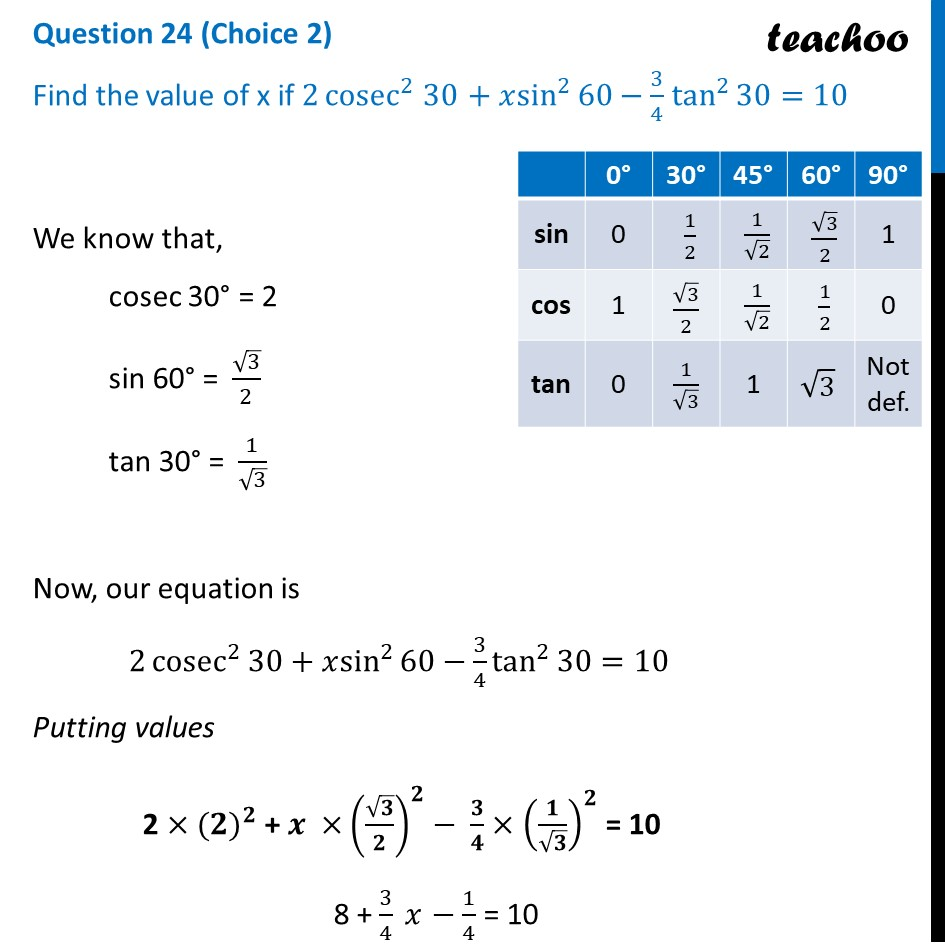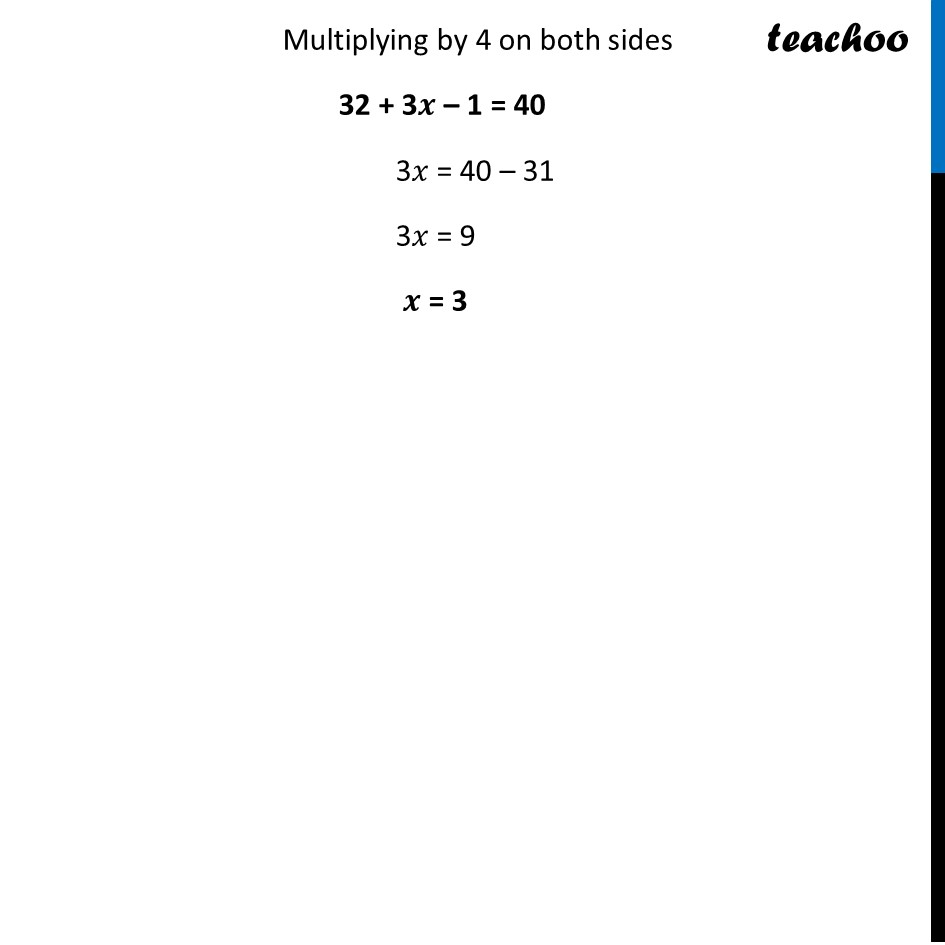CBSE Class 10 Sample Paper for 2024 Boards - Maths Standard

Class 10
Solutions of Sample Papers for Class 10 Boards

## Find the value of x if 2cosec^2⁡30+xsin^2⁡60-3/4 tan^2⁡30=10

This question is similar to Question 1,(ii) Ex 8.2, Chapter 8 Class 10Learn in your speed, with individual attention - Teachoo Maths 1-on-1 Class

### Transcript

We know that, cosec 30° = 2 sin 60° = √3/2 tan 30° = 1/√3 Now, our equation is 2 cosec^2⁡30+𝑥sin^2⁡60−3/4 tan^2⁡30=10 Putting values 2 × 〖(𝟐)〗^𝟐 + 𝒙 × (√𝟑/𝟐)^𝟐− 𝟑/𝟒 × (𝟏/√𝟑)^𝟐 = 10 8 + 3/4 𝑥 − 1/4 = 10 Multiplying by 4 on both sides 32 + 3𝒙 – 1 = 40 3𝑥 = 40 – 31 3𝑥 = 9 𝒙 = 3